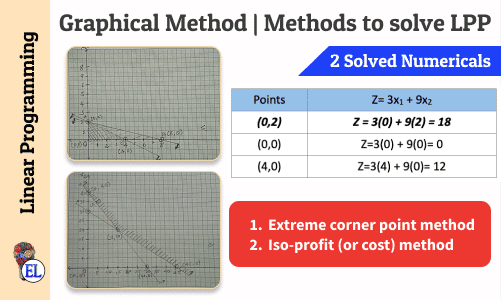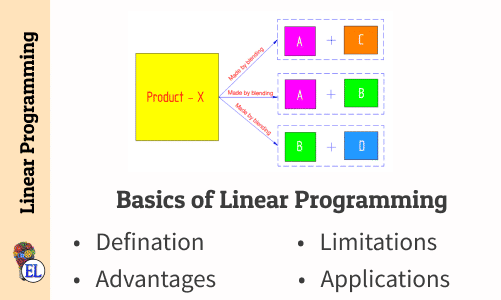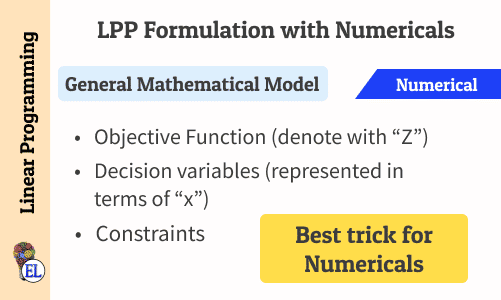Transportation Model

Numerical
Modified Distribution Method (MODI) | Transportation Problem | Transportation Model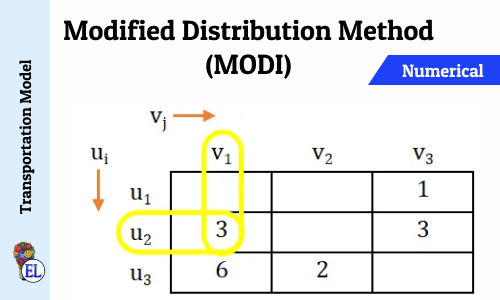Numerical
Stepping Stone | Transportation Problem | Transportation Model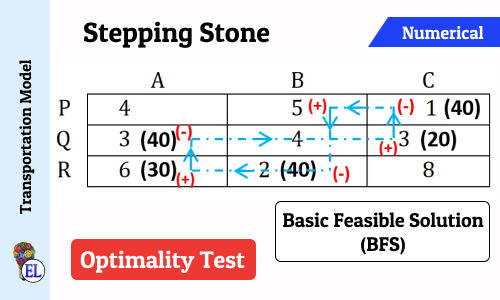Numerical
Vogel’s Approximation Method (VAM) | Method to Solve Transportation Problem | Transportation Model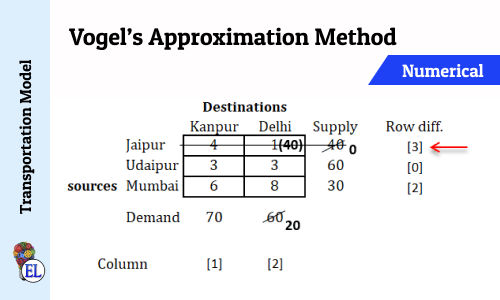Transportation Model - Introduction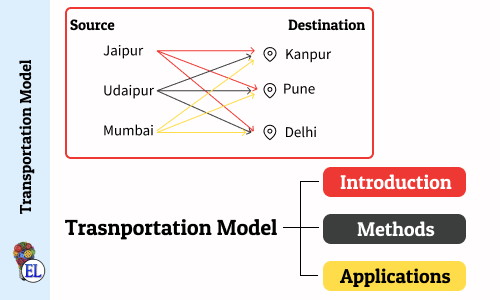Numerical
North West Corner Method | Method to Solve Transportation Problem | Transportation Model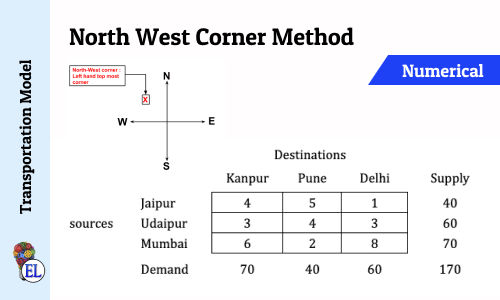Numerical
Least Cost Method | Method to Solve Transportation Problem | Transportation Model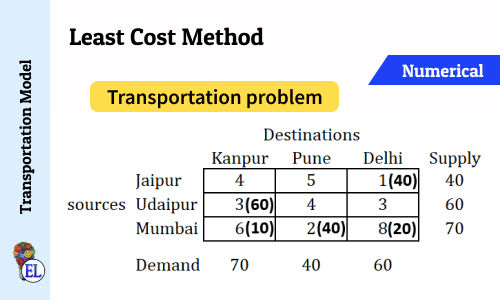Numerical
Tie in selecting row and column (Vogel's Approximation Method - VAM) | Numerical | Solving Transportation Problem | Transportation Model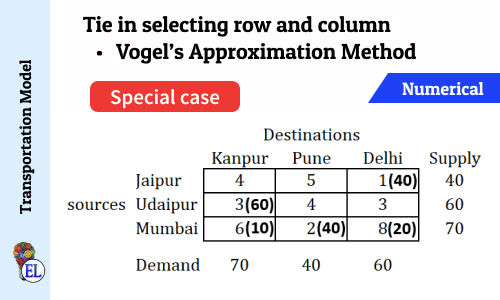Assignment Model

Introduction
Assignment Model | Linear Programming Problem (LPP) | Introduction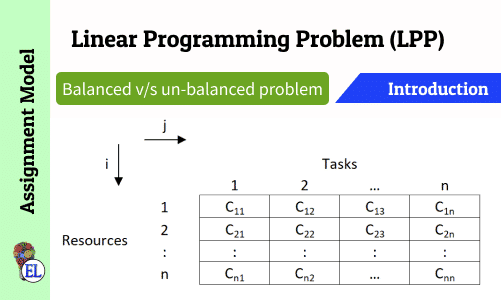Network Analysis

Crashing Special Case - Multiple (Parallel) Critical Paths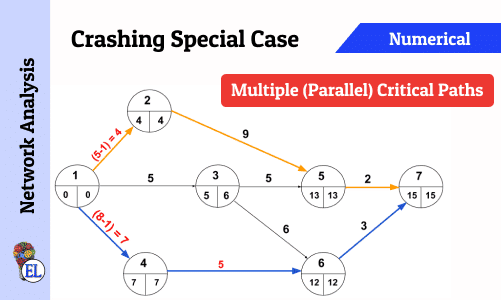Crashing Special Case - Indirect cost less than Crash Cost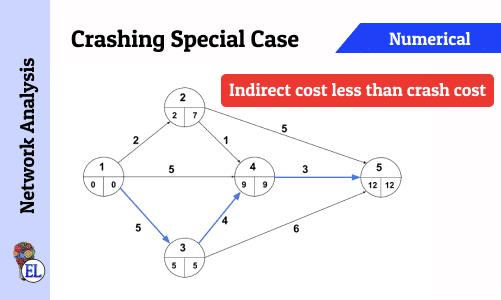Basics of Program Evaluation and Review Technique (PERT)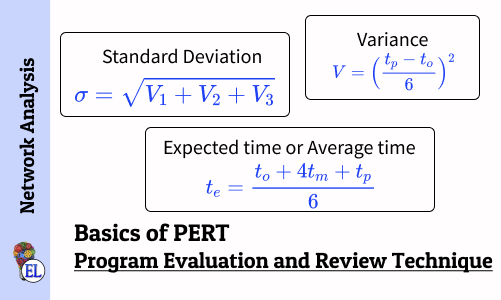Numerical
Numerical on PERT (Program Evaluation and Review Technique)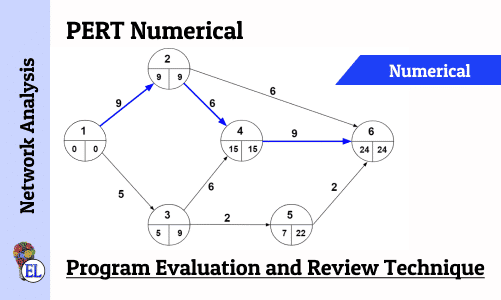Network Analysis - Dealing with Network Construction Basics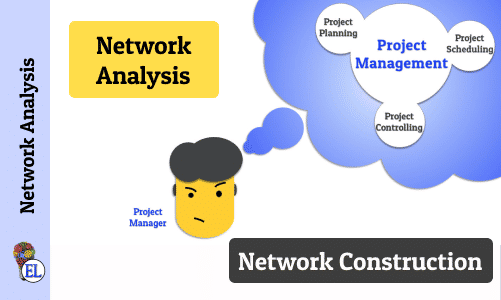Construct a project network with predecessor relationship | Operation Research | Numerical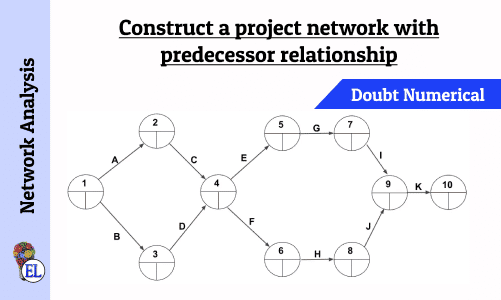Linear programming

2 Solved Numerical
Graphical Method | Methods to solve LPP | Linear Programming